## Indicators

•Cited by SciELO
•Access statistics

•Cited by Google
•Similars in SciELO
•Similars in Google

## Print version ISSN 1794-9165

### ing.cienc. vol.14 no.27 Medellín Jan./June 2018

#### http://dx.doi.org/10.17230/ingciencia.14.27.2

Original articles

Symmetry and Reversibility Properties for Quantum Algebras and Skew Poincaré Birkhoff-Witt Extensions

Propiedades de simetría y reversibilidad para álgebras cuánticas y extensiones torcidas de Poincaré-Birkhoff-Witt

Armando Reyes1  , Julio Jaramillo2

1Universidad Nacional de Colombia, mareyesv@unal.edu.co, https://orcid.org/0000-0002-5774-0822, Bogotá, Colombia.

2Universidad Nacional de Colombia, jcjaramilloq@unal.edu.co, Orinoquia, Colombia.

Abstract

Our aim in this paper is to investigate symmetry and reversibility properties for quantum algebras and skew PBW extensions. Under certain conditions we prove that these properties transfer from a ring of coefficients to a quantum algebra or skew PBW extension over this ring. In this way we generalize several results established in the literature and consider algebras which have not been studied before. We illustrate our results with remarkable examples of theoretical physics.

Key words: Symmetry; reversibility; quantum algebra; skew Poincaré-Birkhoff-Witt extension

Resumen

Nuestro propósito en este artículo es investigar las propiedades de simetría y reversibilidad para álgebras cuánticas y extensiones PBW torcidas. Bajo ciertas condiciones mostramos que estas propiedades se transfieren de un anillo de coeficientes a un álgebra cuántica o extensión PBW torcida sobre este anillo. De esta manera generalizamos diversos resultados establecidos en la literatura, y los ampliamos a álgebras antes no estudiadas. Ilustramos nuestros resultados con ejemplos destacados de la física teórica.

Palabras-clave: Simetría; reversibilidad; álgebra cuántica; extensión torcida de Poincaré-Birkhoff-Witt

1 Introduction

Two of the three concepts of interest in this paper are symmetry and re- versibility. A ring B is called symmetric, if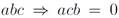for every elements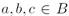. B is said to be reversible, if ab = 0 implies ba = 0, for each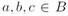(this notion was introduced by Lambek in ). Note that symmetric implies reversible. Anderson and Camillo , Theorem 1.3, proved that reduced rings are symmetric (B is called reduced if B has no nonzero nilpotent elements). Of course, commutative rings are symmetric. However, polynomial rings over reversible rings need not to be reversible, and polynomial rings over symmetric rings need not to be symmetric (c.f.  and  for adequate examples of these assertions). The third concept of interest for us in this article is Armendariz ring. Rege and Chhawchharia  introduced the notion of Armendariz ring B, if for

elements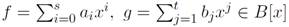which satisfy fg = 0, then

aibj = 0, for every i, j. Since then, the term Armendariz was used inclusive by Armendariz itself  who proved that a reduced ring satisfies this condition.

With the aim of studying noncommutative polynomial versions of symmetric, reversibility and Armendariz in the context of Ore extensions defined by Ore , several notions have been considered in the literature:

(1) rigid ring introduced by Krempa : an endomorphism σ of a ring B is called to be σ-rigid, if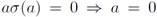, for every a B. B is called σ-rigid, if there exists a rigid endomorphism σ of B. One can prove that any rigid endomorphism is injective and σ-rigid rings are reduced (see Hong et al. ). Different properties of σ-rigid rings have been studied in the literature (see  and  for a detailed list of works). (2) σ-skew Armendariz defined by Hong et al. : if σ is an endomorphism of a ring B, then B is called to be σ-skew Armendariz, if whenever polynomials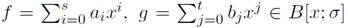With fg = 0, then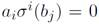, for every i, j. (3) (-Armendariz introduced by Hong et. al in : if ( is an endomorphism of a ring B, then B is called to be (-Armendariz, if for elements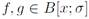given by, respectively,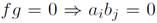, for each, i, j. (4) (σ, δ)-skew Armendariz defined by Hashemi and Moussavi : for elements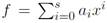and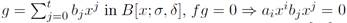, for each, i, j. (5) (σ, δ)-compatible defined by Hashemi and Moussavi : a ring B is (-compatible, if for each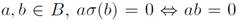; B is said to be δ-compatible, if for each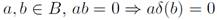. In the case B is both σ-compatible and δ-compatible, B is said to be (σ, δ)-compatible. It is known that B is σ-rigid if and only if B is (σ, δ)-compatible and reduced (, Lemma 2.2). (6) σ-reversible introduced by Baser et. al : B is called right (resp. left) σ-reversible, if whenever ab = 0 we have bσ(a) = 0 (resp. σ(b)a = 0), for a, b B. (7) σ-symmetric defined by Kwak : B is called right (resp. left) σ-symmetric, if whenever abc = 0 implies acσ(b) = 0 (resp. σ(b)ac = 0).

Now, a few years ago we have been studying several ring and module theoretical properties of noncommutative rings more general than Ore ex- tensions of injective type (i.e., when σ is injective). These rings are the skew Poincaré-Birkhoff-Witt extensions (PBW for short), also known as σ-PBW extensions, which were introduced by Gallego and Lezama in  (see , or  for a list of noncommutative rings which are skew PBW extensions but not Ore extensions). Besides of Ore extensions, skew PBW extensions generalize several families of noncommutative rings, and include remarkable algebras appearing in theoretical physics, representation theory, Hopf algebras and quantum groups. Let us see some examples (a detailed reference of every example can be found in ,, or ). (i) universal enveloping algebras of finite dimensional Lie algebras; (ii) PBWextensions introduced by Bell and Goodearl; (iii) almost normalizing extensions defined by McConnell and Robson; (iv) solvable polynomial rings introduced by Kandri-Rody and Weispfenning; (v) diffusion algebras studied by Isaev, Pyatov, and Rittenberg; (vi) 3-dimensional skew polynomial algebras introduced by Bell and Smith; (vii) the regular graded algebras studied by Kirkman, Kuzmanovich, and Zhang, and other noncommutative algebras of polynomial type. The importance of skew PBW extensions is that the coefficients do not necessarily commute with the variables, and these coefficients are not necessarily elements of fields (see Definition 2.1 below). In fact, skew PBW extensions contain well-known groups of algebras such as some types of G-algebras studied by Levandovskyy and some PBW algebras defined by Bueso et. al., (both G-algebras and PBW algebras take coefficients in fields and assume that coefficientes commute with variables), Auslander-Gorenstein rings, some Calabi-Yau and skew Calabi- Yau algebras, some Artin-Schelter regular algebras, some Koszul algebras, quantum polynomials, some quantum universal enveloping algebras, and others (c.f. ,, and ). As we can appreciated, skew PBW extensions include a lot of noncommutative rings much more general than Ore extensions.

Having in mind the results above about the interaction between symmetric, reversible and Armendariz properties over Ore extensions, we consider important to extend all these results to the context of skew PBW extensions. In this way we continue our work of generalizing several results in the literature for Ore extensions. As a matter of fact, the notions (1), (2), (3), (4) and (5) above mentioned have been investigated for skew PBW extensions in ,,, and , respectively, so only the notions (6) and (7) have not considered. It is precisely the purpose of this paper to investigate these notions in the context of skew PBW extensions with a view toward quantum algebras. In this way, we formulate new results for several non-commutative algebras which can not be expressed as Ore extensions, and generalize several results established in papers as ,,,, and , all of them exclusively for Ore extensions.

The paper is organized as follows. In Section 2 we recall some preliminary results about skew PBW extensions. Section 2.1 contains some results about the relation between skew PBW extensions, Σ-rigid rings, (Σ, ∆)- Armendariz rings, Σ-skew Armendariz rings, Σ-Armendariz and (Σ, ∆)- compatible rings. There, we introduce the condition ( Σ) (Definition 2.9) which is very important in the next section. Precisely, Section 3 contains the original results of the paper. We characterize reversibility and symmetry properties over quantum algebras and skew PBW extensions (Theorem 3.1), and then we introduce our definitions of right (resp. left) Σ-reversible and right (resp. left) Σ-symmetric (Definition 3.1) which extends notions (6) and (7) mentioned above. In this way, the results presented in this section (Theorems 3.2, 3.3, 3.4) are new for skew PBW extensions and quantum algebras, and all of them extend similar results for Ore extensions appearing in ,,,, and . Then, in Section 4 we present important quantum algebras appearing in theoretical physics, which are examples of skew PBW extensions and satisfy the results described in Section 3. Finally, in Section 5 we make some comments about a future work concerning algebraic characterizations of objects appearing in theoretical physics.

Throughout the paper, the word ring means a ring (not necessarily commutative) with unity. C will denote the field of complex numbers and the letter k will denote any field.

2 Skew PBW extensions

In this section we recall some results about skew PBW extensions which are important for the rest of the paper.

Deftnition 2.1 (, Definition 1). Let R and A be rings. We say that A is a skew PBW extension (also known as σ-PBW extension) of R, which is denoted by A := σ(R)(x1, . . . , xn), if the following conditions hold:

(i) R ⊆ A;

(ii) there exist elements x1, . . . , xn ∈ A such that A is a left free R- module, with basis Mon(A) := {xα = xα1 · · · xαn | α = (α1, . . . , αn) ∈

n

(iii) For each 1 ≤ i ≤ n and any r ∈ R \ {0}, there exists an element

ci,r ∈ R \ {0} such that xir − ci,rxi ∈ R.

(iv) For any elements 1 ≤ i, j ≤ n, there exists ci,j ∈ R \ {0} such that

xjxi − ci,jxixj ∈ R + Rx1 + · · · + Rxn.

Since Mon(A) is a left R-basis of A, the elements ci,r and ci,j are unique (, Remark 2).

Proposition 2.1 (, Proposition 3). Let A be a skew PBW extension of R. For each 1 ≤ i ≤ n, there exist an injective endomorphism σi : R → R and an σi-derivation δi : R → R such that xir = σi(r)xi+δi(r), for each r ∈ R. From now on, we will write Σ := {σ1, . . . , σn}, and ∆ := {δ1, . . . , δn}.

Deftnition 2.2 (, Definition 4 and , Definition ). Letx A be a skew PBW extension of R.

1. A is called quasi-commutative if the conditions (iii) and (iv) in Definition 2.1 are replaced by the following: (iii’) for each 1 ≤ i ≤ n and all r ∈ R \ {0}, there exists ci,r ∈ R \ {0} such that xir = ci,rxi; (iv’) for any 1 ≤ i, j ≤ n, there exists ci,j ∈ R \ {0} such that xjxi = ci,jxixj.

2. A is called bijective, if σi is bijective for each 1 ≤ i ≤ n, and ci,j is invertible, for any 1 ≤ i < j ≤ n.

3. A is called of endomorphism type, if δi = 0, for every i. In addition, if every σi is bijective, A is a skew PBW extension of automorphism type.

Example 2.1. (, p. 1212). If R[x1; σ1, δ1] [xn; σn, δn] is an iterated Ore extension where

• σi is injective, for 1 ≤ i ≤ n;

• σi(r), δi(r) ∈ R, for every r ∈ R and 1 ≤ i ≤ n;

• σj(xi) = cxi + d, for i < j, and c, d ∈ R, where c has a left inverse;

• δj(xi) ∈ R + Rx1 + · · · + Rxn, for i < j,

then R[x1; σ1, δ1] [xn; σn, δn] ∼= σ(R) (x1, . . . , xn( . In general, skew PBW extensions are more general than Ore extensions of injective type (see  for different examples which are skew PBW extensions but can not be expressed as iterated Ore extensions).

Deftnition 2.3. If A is a skew PBW extension of R, then:

(i) for α = (α1, . . . , αn) ∈ Nn, σα := σα1 · · · σαn , |α| := α1 + · · · + αn. If

β = (β1, . . . , βn) ∈ Nn, then α + β := (α1 + β1, . . . , αn + βn).

(ii) For X = xα ∈ Mon(A), exp(X) := α, deg(X) := |α|, and X0 := 1. The symbol ⪰ will denote a total order defined on Mon(A) (a total order on Nn). For an element xα ( Mon(A), exp(xα) := α ( Nn. If xα ⪰ xβ but xα ( xβ, we write xα ≻ xβ. Every element f ( A can be expressed uniquely as f = a0 + a1X1 + + amXm, with ai ( R, and Xm ≻ ≻X1 (eventually, we will use expressions as f = a0 + a1Y1 + + amYm, with ai R, and Ym≻ ≻Y1). With this notation, we define lm(f ) := Xm, the leading monomial of f ; lc(f ) := am, the leading coefficient of f ; lt(f ) := amXm, the leading term of f ; exp(f ) := exp(Xm), the order of f ; and E(f ) := {exp(Xi) | 1 ≤ i ≤ t}. Note that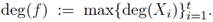.

Finally, if f = 0, then lm(0) := 0, lc(0) := 0, lt(0) := 0. We also consider X ≻0 for any X Mon(A). For a detailed description of monomial orders in skew PBW extensions, see , Section 3.

Proposition 2.2 (, Proposition 2.9). If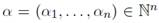and r is an element of R, then

Remark 2.1 (, Remark 2.10 (iv)). About Proposition 2.2, we have the following observation: if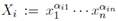and ,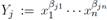, when we compute every summand of aiXibjYj we obtain products of the coefficient ai with several evaluations of bj in σ’s and δ’s depending of the coordinates of αi. This assertion follows from the expression:

2.1 Σ-rigid rings and some generalizations

In this section we recall several Armendariz notions and their relation with skew PBW extensions.

Deftnition 2.4 (, Definition 3.2). Let B be a ring and Σ a family of endomorphisms of B. Σ is called a rigid endomorphisms family, if rσα(r) = 0 implies r = 0, for every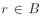and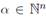. A ring B is called to be Σ-rigid, if there exists a rigid endomorphisms family Σ of B.

Note that if Σ is a rigid endomorphisms family, then every element σi ( Σ is a monomorphism. In fact, Σ-rigid rings are reduced rings: if B is a Σ-rigid ring and r2 = 0 for r ( B, then we have the equalities 0 = rσα(r2)σα(σα(r)) = rσα(r)σα(r)σα(σα(r)) = rσα(r)σα(rσα(r)), i.e., rσα(r) = 0 and so r = 0, that is, B is reduced (note that there exists an endomorphism of a reduced ring which is not a rigid endomorphism, see  for more details). With this in mind, we consider the family of injective endomorphisms Σ and the family ∆ of Σ-derivations in a skew PBW extension A of a ring R (see Proposition 2.1).

Several notions of Armendariz ring have been defined with the aim of generalizing the concept of Σ-rigid ring.

Deftnition 2.5 (, Definition 3.4). Let A be a skew PBW extension of a ring R. We say that R is an (Σ, ∆)-Armendariz ring, if for polynomials f = a0 + a1X1 + + amXm and g = b0 + b1Y1 + + btYt in A, the equality fg = 0 implies aiXibjYj = 0, for every i, j.

Deftnition 2.6. (, Definition 3.1) Let A be a skew PBW extension of a ring R. R is called a Σ-skew Armendariz ring, if for elements f =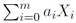and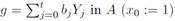, the equality fg = 0 implies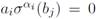, for all, 0 ≤ i ≤ m and 0 ≤ j ≤ t, where

Remark 2.2 (, Remark 3.4). We have the following facts:

Consider the ring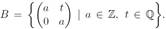Then B is a commutative ring, and if we consider the automorphism σ of R given by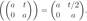. In , Example 1, it was shown that R

is σ-skew Armendariz and is not a σ-rigid. Since Σ-rigid and Σ-skew Armendariz are generalizations of σ-rigid and σ-skew Armendariz, respectively, this example shows that there exist an example of a Σ-skew Armendariz ring which is not Σ-rigid.

Let B = Z2[x] be the commutative polynomial ring over Z2, and σ the endomorphism of B = Z2[x] defined by σ(f (x)) = f (0). Then B = Z2[x] is σ-skew Armendariz and is not σ-rigid (, Example 5).

From definitions above we can establish the following relations

Σ−rigid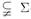−skew Armendariz,

(Σ, ∆)−Armendariz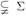−skew Armendariz.

Nevertheless, the above conditions coincide if we consider R as a reduced ring. More precisely,

Proposition 2.3 (, Theorem 3.9, and , Theorem 3.6). If A is a skew PBW extension of a ring R, then the following statements are equivalent:

(i) R is reduced and (Σ, ∆)-Armendariz (resp. Σ-skew Armendariz) (ii) R is Σ-rigid (iii) A is reduced.

Next we introduce Σ-Armendariz rings which extend the notion of σ- Armendariz given by Hong et. al . Since this notion was defined for Ore extensions of the form B[x; σ], i.e., where δ = 0, we consider our notion for skew PBW extensions of endomorphism type.

Deftnition 2.7. Let A be a skew PBW extension of endomorphism type over a ring R. R is called a Σ-Armendariz ring, if whenever polynomials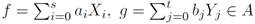satisfy fg = 0, then a i b j = 0, for each i, j.

As another generalization of rigid rings, we have the notion of (Σ, ∆)- compatible ring in the following way.

Deftnition 2.8 (, Definition 3.2). Consider a ring R with a family of endomorphisms Σ and a family of Σ-derivations ∆. Then, (1) R is said to be Σ-compatible, if for each a, bR, α (b) = 0 if and only if ab = 0, for every α ∈ Nn. (2) R is said to be ∆-compatible, if for each a, bR, ab = 0 implies β (b) = 0, for every β (Nn. (3) If R is both Σ-compatible and

∆-compatible, R is called or (Σ, ∆)-compatible.

If we impose the reduceness of the ring R, the notion of rigid ring coincides of the concept of (Σ, ∆)-compatible as the following proposition shows

Proposition 2.4 (, Theorem 3.9). If A is a skew PBW extension of a ring R, then the following statements are equivalent:

1. R is reduced and (Σ, ∆)-compatible;

2. R is Σ-rigid;

3. A is reduced.

From Propositions 2.3 and 2.4 we can see that the concepts of Σ-rigid, (Σ, ∆)-Armendariz, Σ-skew Armendariz and (Σ, ∆)-compatible are equivalent assuming R to be reduced. Now, as we saw in the Introduction, reduced ( symmetric ( reversible (in general, each of these implications is false, see ), so if we want to extend the symmetric and reversibility properties from a ring R to a quantum algebra or skew PBW extension A over R (this is the content of Section 3), it will be crucial not assuming R to be reduced together a condition (C Σ) which is more weak than (Σ, ∆)- compatible ring. This condition is motivated by the analogous condition (C σ ) introduced by Yakoub and Louzari in  for Ore extensions.

Deftnition 2.9. Let B be a ring and Σ a family of endomorphisms of B. We say that B satisfies the condition (CΣ), if the equality α (b) = 0 implies ab = 0, for each elements a, bB and every α ∈ Nn.

Remark 2.3. (i) The family of Σ-rigid rings is strictly contained in the family of (Σ, ∆)-skew Armendariz rings satisfying the condition (C Σ). (ii) The (Σ, ∆)-skew Armendariz property is independent from the condition (C Σ), as we can appreciate in , Examples 1.4 and 1.5. (iii) There exists an example of a ring R which is not Σ-rigid, R satisfies the condition (CΣ), and R is Σ-skew Armendariz, see , Example 1.6. For a detailed discussion about all these notions, see , Section 3.

From Definitions 2.5 and 2.6 we can see that Σ-Armendariz rings are included in Σ-skew Armendariz, but the converse is false, see , Remark 3.4. However, the following results of this section show the importance of the condition (CΣ).

Theorem 2.1. If A is a skew PBW extension of endomorphism type over a ring R, then R is Σ-Armendariz if and only if R is Σ-skew Armendariz and satisfies the condition (CΣ).

Proof. Suppose that R is Σ-Armendariz. Consider two elements f, gA given by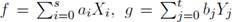with fg = 0. By assumption, a i b j = 0, for each i, j, so ), Proposition 3.8 implies that a i σ α i (b j ) = 0, for every i, j, i.e., R is Σ-skew Armendariz. Again, , Proposition 3.8 asserts that R satisfies the condition (C Σ).

Conversely, consider two elements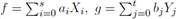of A with fg = 0. We know that a i σ α i (b j ) = 0, for all i, j, and using that R satisfies the condition (C Σ) we obtain that a i b j = 0, for every i, j. Therefore R is Σ-Armendariz.

Corollary 2.1. (, Theorem 2.6). R is σ-Armendariz if and only if R

is σ-skew Armendariz and satisfies the condition (C σ ).

Next we present some key facts which are very useful in the proof of the results established in Section 3. We remark the importance of the condition (CΣ).

Proposition 2.5. If R is a (Σ, ∆)-skew Armendariz ring which satisfies the condition (CΣ), then the equality ab = 0 implies σ α (a)b = δ α (a)b = 0, for all a, bR and α ∈ Nn.

Proof. We only show that ab = 0 ( σ i (a)b = δ i (a)b = 0, for any 1 ≤ in. Consider elements f = σ i (a)x i + δ i (a), g = b ( A, for any i, with ab = 0. Note that fg = σ i (a)x i b+δ i (a)b = σ i (ab)x i +σ i (a)δ i (b)+δ i (a)b = σ i (ab)x i + δ i (ab) = 0. Proposition 2.7 implies that σ i (a)b = δ i (a)b = 0.

Proposition 2.6. If R is an (Σ, ∆)-skew Armendariz and reversible ring satisfying the condition (CΣ), then the equality ab = 0 implies that ax α b = 0, for every a, bR and each α ∈ Nn.

Proof. Consider elements a, bR with ab = 0. Then ba = 0 (R is reversible) whence σ α (b)a = δ α (b)a = 0, for any α ∈ Nn (Proposition 2.5). Hence α (b) = α (b) = 0, for each α Nn, and the assertion follows from Proposition 2.2 ∈ and Remark 2.1.

Proposition 2.7. Let A be a skew PBW extension over a ring R. If R is (Σ, ∆)-skew Armendariz and satisfies the condition (C Σ), then for any elements f, g ∈ given by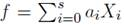and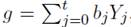, respectively, fg = 0 ⇒ aibj = 0, for all i, j.

Proof. Consider elements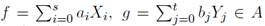such that

fg = 0. By assumption, R is (Σ, ∆)-skew Armendariz, so a i X i b j Y j = 0, for all i, j.

Following Remark 2.1, if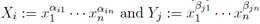,

when we compute every summand of aiXibjYj we obtain products of the coefficient ai with several evaluations of bj in σ’s and δ’s depending of the coordinates of αi, which follows from the expression

Since fg = 0, lc(aiXibjYj) = aiσαi (bj) = 0, for each i, j, the condition (CΣ) implies that aibj = 0, for every i, j, which concludes the proof.

Remark 2.4. Proposition 2.7 establishes that Σ-skew Armendariz rings satisfying the condition ( Σ) are Σ-Armendariz. The condition ( Σ) is very important in this assertion as we can appreciate in , Example 2.2. More precisely, this example shows a Σ-skew Armendariz which is not Σ-Armendariz and does not satisfy the condition (CΣ).

Proposition 2.8. Let A be a skew PBW extension of a (Σ, ∆)-skew Armendariz ring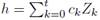are elements of A, then fgh = 0 ( a i b j c k = 0, for every value of i, j, k.

Proof. Let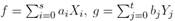and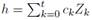be elements of A with fgh= 0 we obtain ai(gh) = 0 for each i, whence (aig)h = 0, for all i, so Proposition 2.7 guarantees that a i b j c k = 0, for all values i, j, k.

3 Reversibility, symmetry, Σ-reversibility and Σ-symmetry

In this section we characterize reversibility, symmetry, Σ-reversibility and Σ-symmetry properties of quantum algebras and skew PBW extensions.

Theorem 3.1. Let A be a skew PBW extension over a (Σ, ∆)-skew Armendariz ring R which satisfies the condition (CΣ). Then:

R is reversible if and only A is reversible.

R is symmetric if and only if A is symmetric.

Proof. First of all, note that a subring of a symmetric (resp. reversible) ring is symmetric (resp. reversible). Let us prove (1). Consider elements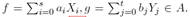If fg = 0, then a i b j = 0, for all i, j (Proposition 2.7), and using that R is reversible we obtain b j a i = 0, for each i, j. In this way, Proposition 2.6 guarantees that b j Y j a i X i = 0, for all i, whence gf = 0.

of A. If fgh = 0, then a i b j c k = 0, for all i, j, k (Proposition 2.8. Having in mind that R is symmetric, a i c k b j = 0, for each i, j, k, and since R is reversible, a i (c k Z k b j Y j ) = 0, for every i, j, k, by Proposition 2.6, so a i X i c k Z k b j Y j = 0, for all i, j, k. Therefore fgh = 0.

Corollary 3.1. Let A be a skew PBW extension over a (Σ, ∆)-skew Armendariz ring R which is (Σ, ∆)-compatible. Then:

(1) R is reversible if and only A is reversible.

(2) R is symmetric if and only if A is symmetric.

Corollary 3.2 (, Theorem 2.4). Let R be an (σ, δ)-skew Armendariz ring satisfying the condition (C σ ). Then (1) R is reversible if and only if R[x; σ, δ] is reversible. (2) R is symmetric if and only if R[x; σ, δ] is symmetric.

Corollary 3.3. (, Theorem 3.6). Let B be an σ-Armendariz ring. Then B is reversible if and only if B[x; σ] is reversible. (2) B is symmetric if and only if B[x; σ] is symmetric. (, Proposition 3.4 and , Proposition 2.4). Let B be an Armendariz ring. Then (1) B is reversible if and only if B[x] is reversible. (2) B is symmetric if and only if B[x] is symetric.

Now, in , Baser et. al defined a ring B as right (resp. left) σ- reversible, if whenever ab = 0, for a, b (R, (a) = 0 (resp. σ(b)a = 0). On the other hand, Kwak  called a ring B right (resp. left) σ-symmetric, if whenever abc = 0, for a, b, c (R, acσ(b) = 0 (resp. σ(b)ac = 0). With the aim of extending these notions in a natural way for the class of skew PBW extensions, we present the following definition.

Deftnition 3.1. Let B be a ring and Σ = (σ 1 , . . . , σ n ( a family of endomorphisms of B. B is called right (resp. left) Σ-reversible, if whenever ab = 0, for a, b ( R, i (a) = 0 (resp. σ i (b)a = 0), for all i. B is called right (resp. left) Σ-symmetric, if whenever abc = 0, for a, b, c ( R, acσ i (b) = 0 (resp. σ i (b)ac = 0), for each i.

Theorem 3.2. Let R be a ring and Σ a family of endomorphisms of R. If

R satisfies the condition (CΣ), then:

(1) R is reversible if and only if R is Σ-reversible.

(2) R is symmetric if and only if R is Σ-symmetric.

Proof. (1) Consider two elements a, b ( B with ab = 0 ( i (a) = 0, for some fixed i. Using the condition (C Σ), we obtain ba = 0, i.e., R is reversible. Conversely, let a, b be elements of R with ab = 0. If R is not right reversible we have that i (a) ( 0, and since R is reversible, σ i (a)b ( 0. Having in mind that R satisfies the condition (C Σ), σ i (ab) = 0 which contradicts that σ i (ab) = 0. On the other hand, if σ i (b)a ( 0, that is, R is not left σ-reversible, the condition (C Σ) guarantees that σ i (ba) ( 0, and again we obtain a contradiction (R is reversible).

(2) Consider elements a, b, c (R with abc = 0. It follows that bca = 0 (R is reversible), whence caσ i (b) = 0 (R is right σ-reversible), so σ i (b)ca = 0 (again, R is reversible), for some fixed i, and then σ i (b)ac = 0 which shows that R is left Σ-symmetry. Using a similar reasoning one can prove that R is right Σ-symmetry. Finally, if abc = 0, using the right Σ-symmetry we obtain that acσ i (b) = 0, and so acb = 0 (R satisfies the condition (CΣ).

Corollary 3.4. If R is a (Σ, ∆)-compatible ring, then:

(1) R is reversible if and only if R is Σ-reversible.

(1) R is symmetric if and only if R is Σ-symmetric.

Corollary 3.5 (, Lemma 3.1). Let R be a ring and σ an endomorphism of R. If R satisfies the condition (C σ ), then

(1) R is reversible if and only if R is σ-reversible.

(2) R is symmetric if and only if R is σ-symmetric.

As the following examples shows, the condition (C Σ) is completely necessary in Proposition 3.2.

Example 3.1 (, Example 3.2). Let Z2 be the ring of integers modulo 2, and consider R: = Z2 (Z2 with the usual addition and multiplication. It is easy to see that R is commutative, and so symmetric and reversible. If we take the (injective) endomorphism σ of R given by σ((a, b)) = (b, a), then (1) R is not σ-reversible (and hence not σ-symmetric), since (1, 0)(0, 1) = 0 but (0, 1)σ((1, 0)) = (0, 1)(0, 1) = (0, 1) ƒ= 0. (2) R does not satisfy the condition (C Σ), since (1, 0)σ((1, 0)) = 0 but (1, 0)2 = (1, 0) ƒ= 0,

Theorem 3.3. If A is a skew PBW extension over an (Σ, ∆)-skew Armendariz ring which satisfies the condition (C Σ), then the following statements are equivalent: (i) R is reversible (ii) R is Σ-reversible (iii) R is right Σ- reversible (iv) A is reversible.

Proof. (i) ⇔ (iv) Theorem 3.1. (i) ⇒ (ii) and (ii) ⇒ (iii) Theorem 3.2. (iii) ⇒ (i) Consider elements a, bR with ab = 0 ⇒ i (a) = 0, for every i = 1, . . . , n. From the condition (CΣ), it follows that ba = 0.

Corollary 3.6. If A is a skew PBW extension over an (Σ, ∆)-skew Armendariz ring which is (Σ, ∆)-compatible, then the following statements are equivalent: (i) R is reversible (ii) R is Σ-reversible (iii) R is right Σ- reversible (iv) A is reversible.

Theorem 3.4. If A is a skew PBW extension over an (Σ, ∆)-skew Armendariz ring which satisfies the condition (C Σ), then the following statements are equivalent: (i) R is symmetric (ii) R is Σ-symmetric (iii) R is right Σ-symmetric (iv) A is symmetric.

Proof. The arguments are similar to the used in the proof of Theorem 3.3.

Corollary 3.7. If A is a skew PBW extension over an (Σ, ∆)-skew Armendariz ring which is (Σ, ∆)-compatible, then the following statements are equivalent: (i) R is symmetric (ii) R is Σ-symmetric (iii) R is right Σ-symmetric (iv) A is symmetric.

The following facts established in the literature are consequences of our theorems above.

Corollary 3.8 (, Theorem 3.3). Let R be an (σ, δ)-skew Armendariz ring satisfying the condition (C σ ). Then R is reversible ⇔ R is σ-reversible. ⇔ R is right σ-reversible ⇔ R[x; σ, δ] is reversible.

(, Theorem 3.4). Let B be an (σ, δ)-skew Armendariz ring satisfying the condition (C σ ). Then: B is symmetric ⇔ B is σ-symmetric ⇔ B is right σ-symmetric ⇔ B[x; σ, δ] is symmetric.

(, Corollary 2.11). Let B be an σ-Armendariz ring. Then: B is reversible (B is σ-reversible (B is right σ-reversible (B[x; σ] is reversible.

(, Theorem 2.10). Let B be an σ-Armendariz ring. Then: B is symmetric (B is σ-symmetric (B is right σ-symmetric (B[x; σ] is symmetric.

4 Examples of quantum algebras

In this section we present quantum algebras of interest in theoretical physics. All these algebras are examples of skew PBW extensions (see  and  for a proof of this assertion), so the results established in Section 3 can be applied to one of them. The detailed references of every algebra can be found in ,, or .

(1) Weyl algebra. The first Weyl algebra A 1(k) over k is defined to be the k-algebra generated by the indeterminates x, y subject to the relation yx = xy + 1. The nth Weyl algebra A n (k) over k is the k-algebra generated by the 2n indeterminates x 1 , . . . , x n , y 1 , . . . , y n where

x j x i = x i x j , y j y i = y i y j ,1 ≤ i < jn,

y j x i = x i y j + δ ij , δ ij is the Kronecker delta,1 ≤ i, jn. (1)

In the literature, the Weyl algebra is known as the first quantum algebra.

(2) Additive analogue of the Weyl algebra. By definition, the k-algebra A n (q 1 , . . . , q n ) is generated over k by the indeterminates

x 1 , . . . , x n , y 1 , . . . , y n subject to the relations

x i x j = x j x i , y i y j = y j y i ,1 ≤ i < jn,

y i x i = q i x i y i + 1,1 ≤ in,

x j y i = y i x j , i ƒ= j,(2)

where q i ∈ k \ {0}, for every i.

(3) Multiplicative analogue of the Weyl algebra. This k-algebra n (λ ij )

is generated by the indeterminates x 1 , . . . , x n subject to the relations

x j x i = λ ij x i x j , 1 ≤ i < jn, λ ij ∈ k \ {0}.(3)

If n = 2, then O2(λ 21) is the quantum plane. Now, if λ ji = q −2 ƒ( 0, for some element q ( k \ (0( , and every 1 ≤ i < jn, then O n (λ ji ) is the coordinate ring of the so called quantum affine n-space.

(4) Quantum Weyl algebra. This quantum Weyl algebra was introduced with the purpose of studying the Jordan Hecke symmetry. This non- commutative ring can be viewed as a quantization of the usual second Weyl algebra. By definition, A 2(J a,b ) is the k-algebra generated by the indeterminates x 1 , x 2 , ∂ 1 , ∂ 2, with relations (depending on para- meters a, b ∈ k) given by

x 1 x 2 = x 2 x 1 + ax 2 , ∂ 2 1 = 1 2 + b∂ 2

1 2

1 x 1 = 1 + x 1 1 + ax 1 2 , ∂ 1 x 2 = −ax 1 1abx 1 2 + x 2 1 + bx 2 2

2 x 1 = x 1 2 , ∂ 2 x 2 = 1 − bx 1 2 + x 2 2 .(4)

Over any k, if a = b = 0, then A 2(J 0,0 ) ∼= A 2, the usual second Weyl algebra.

(5) q-Heisenberg algebra. This algebra has its root in q-calculus, and it was studied in . By definition, it is the k-algebra h n (q) generated over k by the indeterminates x i , y i , z i , for 1 ≤in, subject to the relations

x i x j = x j x i , y i y j = y j y i , z j z i = z i z j ,1 ≤ i < jn, x i z i qz i x i = z i y i qy i z i = x i y i q −1 y i x i + z i = 0,1 ≤ in,

x i y j = y j x i , x i z j = z j x i , y i z j = z j y i , i( j,

(5)

where q ∈ k \ {0}.

(6) Lie-deformed Heisenberg. By definition, this C-algebra is defined by the commutation relations

q j (1 + jk )p k p k (1 − jk )q j = ikδ jk

[q j , q k ] = [p j , p k ] = 0, j, k = 1, 2, 3,

where q j , p j are the position and momentum operators, and λ jk = λ k δ jk , with λ k real parameters. If λ jk = 0 one recovers the usual Heisenberg algebra.

(7) Hayashi algebra. With the purpose of obtaining bosonic representations of the Drinfield-Jimbo quantum algebras, Hayashi  considered the algebra U which by definition is the C-algebra generated by the indeterminates ω 1 , . . . , ω n , ψ 1 , . . . , ψ n , ψ1∗, . . . , ψn∗ , with relations

ψ j ψ i ψ i ψ j = ψjψi∗ − ψiψj∗ = ω j ω i ω i ω j = ψjψ i ψ i ψj∗ = 0,1 ≤ i < jn, ω j ψ i q −δ ij ψ i ω j = ψjω i q −δ ij ω i ψj∗ = 0,1 ≤ i, jn,

ψiψ i q 2 ψ i ψi∗ = − q 2 ω 2 , Aˆ q ∈ C 1 ≤ in.

(8) Non-Hermitian realization of a Lie deformed. Following , this C-algebra is an important example of a non-canonical Heisenberg algebra considering the case of non-Hermitian (i.e., k = 1) operators A j , B k , where the following relations are satisfied:

and,

with A j ( A j + , B k ( B k + (j, k = 1, 2, 3). If the operators A j , B k are in the form A j = f j (N j + 1)a j , B k = a k + f k (N k + 1), where a j , a j + are leader operators of the usual Heisenberg-Weyl algebra, with N j the corresponding number operator (N j = a j + a j , N j ( n j ( = n j ( n j ( ), and the structure functions f j (N j + 1) complex, then it is showed that A j and B k are given by

(9) Complex algebra. Let q (C such that q 8 ( 1. The complex algebra V q (sl3(C)) is the C-algebra generated by the indeterminates e 12 , e 13 , e 23, f 12 , f 13 , f 23 , k 1 , k 2 , l 1 , l 2 subject to the following relations:

(10) Diffusion algebras. These algebras arose in physics as a possible way to understand a large class of 1-dimensional stochastic process. A diffusion algebra A with parameters a ij ∈ C \ {0}, 1 ≤ i, jn is an algebra over C generated by variables x 1 , . . . , x n subject to relations a ij x i x j b ij x j x i = r j x i r i x j , whenever i < j, b ij , r i C, for all i < j. In the applications to physics the parameters a ij are strictly positive reals and the parameters b ij are positive reals as they are unnormalised measures of probability. Under certain conditions on the coefficients, some of these algebras are skew PBW extensions.

5 Conclusions

As it was said in , p. 55, “Historically, the importance of quantum algebras has been considered for several authors in the context of quantum mechanics. They have presented purely algebraic formulations of quantum mechanics which does not require the specification of a space of state vectors; rather, the required vector spaces can be identified as substructures in the algebra of dynamical variables (suitably extended for bosonic systems) [...] From a philosophical point of view, it is very important the new relationships between physics and mathematics that emerge with Heisen- berg’s discovery of matrix mechanics and its development in the work of Born, Jordan, and Heisenberg himself. Precisely, this is the Einstein’s view of the Heisenberg method, as “a purely algebraic method of description of nature” ”. On the other hand, the algebraic approach in theoretical physics has been also considered for a lot of physics and mathematicians as a possible reconciliation of the quantum mechanics with general relativity theory, where the gravity does not need to be quantized (c.f. ).

With all this in mind, the characterization of ring theoretical properties of noncommutative algebras for the study of physical objects is a current topic of research. A lot of works have been published in this direction (as we can appreciate with a quick search in the web), and the present paper wants to contribute to this end. If we (and the other authors) are right, then possible questions for a future work can be formulated in terms of homological and cohomological invariants of noncommutative objects (for instance ). Of course, it is impossible to formulate here all possible questions, so we only say that our work continues with the search of algebraic, geometric and analytic properties of this kind of objects.

Acknowledgements

The first author is supported by Grant HERMES Code 41535, Departa- mento de Matemáticas, Universidad Nacional de Colombia, Sede Bogotá.

References

1 J. Lambek, “On the representation of modules by sheaves of factor modules,” Canad. Math. Bull., vol. 14, pp. 359-368, 1971. 30 [ Links ]

2. D. Anderson and V. Camillo, “Semigroups and rings whose zero products commute,” Commun. Algebra, vol. 27, no. 6, pp. 2847-2852, 1999. 30 [ Links ]

3. N. K. Kim and Y. Lee, “Extensions of reversible rings,” J. Pure Appl. Algebra, vol. 185, pp. 207-223, 2003. 30, 32, 33, 42 [ Links ]

4. Z. Wang and L. Wang, “Polynomial rings over symmetric rings need not to be symmetric,” Commun. Algebra , vol. 34, pp. 1043-1047, 2006. 30 [ Links ]

5. M. B. Rege and S. Chhawchharia, “Armendariz rings,” Proc. Japan. Acad. Ser. A Math. Sci., vol. 73, pp. 14-17, 1997. 30 [ Links ]

6. E. P. Armendariz, “A note on extensions of Baer and p.p.-rings,” J. Australian Math. Soc., vol. 18, pp. 470-473, 1974. 30 [ Links ]

7. O. Ore, “Theory of non-commutative polynomials,” Ann. of Math. (2), vol. 34, no. 3, pp. 480-508, 1933. 30 [ Links ]

8. J. Krempa, “Some examples of reduced rings,” Algebra Colloq., vol. 3, no. 4, pp. 289-300, 1996. 30 [ Links ]

9. C. Y. Hong, N. K. Kim , and T. K. Kwak, “Ore extensions of Baer and p.p.- rings,” J. Pure Appl. Algebra , vol. 151, no. 3, pp. 215-226, 2000. 31 [ Links ]

10. A. Reyes, “Skew PBW extensions of Baer, quasi-Baer, p.p. and p.q.-rings,” Rev. Integr. Temas Mat., vol. 33, no. 6, pp. 173-189, 2015. 31, 32, 35, 36 [ Links ]

11. A. Reyes and H. Suárez, “σ-PBW extensions of skew Armendariz rings,” Adv. Appl. Clifford Algebr., vol. 27, no. 4, pp. 3197-3224, 2017. 31, 32, 37, 39 [ Links ]

12. C. Y. Hong , N. K. Kim , andT. K. Kwak , “On Skew Armendariz Rings,” Commun. Algebra , vol. 31, no. 1, pp. 103-122, 2003. 31, 37 [ Links ]

13. C. Y. Hong , T. K. Kwak , and S. T. Rezvi, “Extensions of generalized Armendariz rings,” Algebra Colloq ., vol. 13, no. 2, pp. 253-266, 2006. 31, 32, 33, 37, 42 [ Links ]

14. E. Hashemi and A. Moussavi, “On (σ, δ)-skew Armendariz rings,” J. Korean Math. Soc., vol. 42, no. 2, pp. 353-363, 2005. 31 [ Links ]

15. E. Hashemi and A. Moussavi , “Polynomial extensions of quasi-Baer rings,” Acta. Math. Hungar., vol. 107, no. 3, pp. 207-224, 2005. 31 [ Links ]

16. M. Baser, C. Y. Hong , andT. K. Kwak , “On extended reversible rings,” Algebra Colloq ., vol. 16, no. 1, pp. 37-48, 2009. 31, 32, 33, 42, 45 [ Links ]

17. T. K. Kwak , “Extensions of extended symmetric rings,” Bull. Korean Math. Soc., vol. 44, pp. 777-788, 2007. 31, 32, 33, 42, 45 [ Links ]

18. C. Gallego and O. Lezama, “Gröbner bases for ideals of σ-PBW extensions,” Commun. Algebra , vol. 39, no. 1, pp. 50-75, 2011. 31, 33, 34, 35 [ Links ]

19. A. Reyes , “Ring and module theoretical properties of skew PBW extensions,” Ph.D. dissertation, Universidad Nacional de Colombia, Sede Bogotá, 2013. 31, 45 [ Links ]

20. O. Lezama andA. Reyes , “Some homological properties of skew PBW ex- tensions,” Commun. Algebra , vol. 42, no. 3, pp. 1200-1230, 2014. 31, 34 [ Links ]

21. A. Reyes , “Jacobson’s conjecture and skew PBW extensions, Rev. Integr. Temas Mat., vol. 32, no. 2, pp. 139-152, 2014. 31. [ Links ]

22. C. Gallego andO. Lezama , “Projective modules and Gröbner bases for skew PBW extensions,” Dissertationes Math., vol. 521, pp. 1-50, 2017. 31 [ Links ]

23. A. Reyes andH. Suárez , “A notion of compatibility for Armendariz and Baer properties over skew PBW extensions,” Rev. Un. Mat. Argent., vol. 59, no. 1, pp. 157-178, 2018. 31, 32, 38, 39, 45 [ Links ]

24. H. L. Suárez and A. O. Reyes, “Some Relations between N-Koszul, Artin- Schelter regular and Calabi-Yau algebras with skew PBW extensions,” Ciencia en Desarrollo, vol. 6, no. 2, pp. 205-213, 2015. 32 [ Links ]

25. H. L. Suárez and A. O. Reyes , “Calabi-Yau property for graded skew PBW extensions,” Rev Colombiana Mat., vol. 51, no. 2, pp. 221-238, 2017. 32 [ Links ]

26. A. Suárez, H. Reyes, “Koszulity for skew PBW extensions over fields,” JP J. Algebra, Number Theory Appl., vol. 39, no. 2, pp. 181-203, 2017. 32 [ Links ]

27. A. Suárez , H. Reyes , “A generalized Koszul property for skew PBW extensions,” Far East J. Math. Sci., vol. 101, no. 2, pp. 301-320, 2017. 32 [ Links ]

28. A. Reyes andH. Suárez , “Armendariz property for skew PBW extensions and their classical ring of quotients,” Rev. Integr. Temas Mat ., vol. 34, no. 2, pp. 147-168, 2016. 32, 37 [ Links ]

29. A. Nino andA. Reyes , “Some ring theoretical properties for skew Poincaré- Birkhoff-Witt extensions,” Bol. Mat. (N.S.), vol. 24, no. 2, pp. 131-148, 2017. 32, 36, 37 [ Links ]

30. C. Huh, H. K. Kim, N. K. Kim , andY. Lee , “Basic examples and extensions of symmetric rings,” J. Pure Appl. Algebra , vol. 202, pp. 154-167, 2005. 32, 33, 42 [ Links ]

31. L. B. Yakoub and M. Louzari, “Ore Extensions of Extended Symmetric and Reversible Rings,” International Journal of Algebra, vol. 3, no. 9, pp. 423- 433, 2009. 32, 33, 38, 39, 41, 42, 43, 44, 45 [ Links ]

32. O. Lezama , J. Acosta, andA. Reyes , “Prime ideals of skew PBW extensions,” Rev. Un. Mat. Argent ., vol. 56, no. 2, pp. 39-55, 2015. 34 [ Links ]

33. A. Reyes , “σ-PBW extensions of skew Π-Armendariz rings,” Far East J. Math. Sci ., vol. 103, no. 2, pp. 401-428, 2018. 38 [ Links ]

34. A. Reyes andH. Suárez , “Bases for quantum algebras and skew Poincaré- Birkhoff-Witt extensions,” Momento, vol. 54, no. 1, pp. 54-75, 2017. 45, 49 [ Links ]

35. A. Reyes and Y. Suárez, “On the ACCP in skew Poincaré-Birkhoff-Witt extensions,” Beitr. Algebra Geom., pp. 3-21, 2018. 45 [ Links ]

36. R. Berger, “The quantum Poincaré-Birkhoff-Witt theorem,” Comm. Math. Physics, vol. 143, no. 2, pp. 215-234, 1992. 46 [ Links ]

37. T. Hayashi, “Q-Analogues of Clifford and Weyl Algebras - Spinor and Os- cillator Representations of Quantum Enveloping Algebras,” Comm. Math. Phys., vol. 127, no. 6, pp. 129-144, 1990. 47 [ Links ]

38. A. Jannussi, A. Leodaris, and R. Mignani, “Non-Hermitian realization of a Lie-deformed Heisenberg algebra,” Phys. Lett. A, vol. 197, no. 3, pp. 187-191, 1995. 47 [ Links ]

39. D. A. Slavnov, “Possibility of reconciling quantum mechanics with general relativity theory,” Theoret. Math. Phys., vol. 171, no. 3, pp. 848-861, 2012. 49 [ Links ]

40. A. Reyes andH. Suárez , “Some remarks about the cyclic homology of skew PBW extensions,” Ciencia en Desarrollo , vol. 7, no. 2, pp. 99-197, 2016. 49 [ Links ]

Received: April 19, 2018; Accepted: June 02, 2018This is an open-access article distributed under the terms of the Creative Commons Attribution License